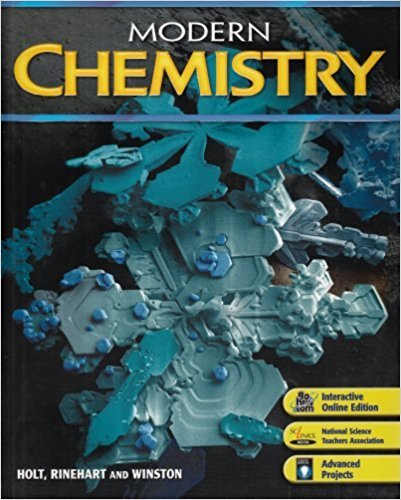×
Log in to StudySoup
Get Full Access to Modern Chemistry - 1 Edition - Chapter 12 - Problem 6
Join StudySoup for FREE
Get Full Access to Modern Chemistry - 1 Edition - Chapter 12 - Problem 6

Already have an account? Login here
×
Reset your password

# a. What is solution equilibrium b. What factors determine the point at which a givenISBN: 9780030735462 452

## Solution for problem 6 Chapter 12

Modern Chemistry | 1st Edition

• Textbook Solutions
• 2901 Step-by-step solutions solved by professors and subject experts
• Get 24/7 help from StudySoup virtual teaching assistantsModern Chemistry | 1st Edition

4 5 1 329 Reviews
15
2
Problem 6

a. What is solution equilibrium? b. What factors determine the point at which a given solute-solvent combination reaches equilibrium?

Step-by-Step Solution:
Step 1 of 3

General Chemistry 202 Professor Kuta Test 2 3 Chapters covered weekly Chapters 46­48 Chapter 46 46.1­ Stoichiometry ­A more in depth review is on noblereaction.org chem 201 text, chapter 19. Below is a quick example for quick review. o Know that with stoichiometry we can calculate G as well as H Example problem:  How many kJ’s can you get from the combustion of 1.000g propane at standard conditions o Okay, kJ’s are not used for enthalpy, but for free energy. (hint: combustion) o Write an equation for this reaction. C 3 8 ) + 5 O (2 

Step 2 of 3

Step 3 of 3

##### ISBN: 9780030735462

Unlock Textbook Solution

Enter your email below to unlock your verified solution to:

a. What is solution equilibrium b. What factors determine the point at which a given